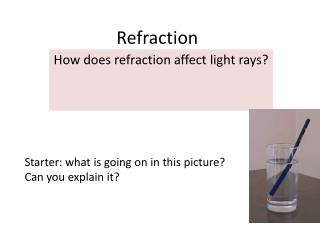DownloadDownload PresentationRefraction

# Refraction

Download Presentation## Refraction

- - - - - - - - - - - - - - - - - - - - - - - - - - - E N D - - - - - - - - - - - - - - - - - - - - - - - - - - -
##### Presentation Transcript

1. Refraction How does refraction affect light rays? Starter: what is going on in this picture? Can you explain it?

2. Demos

3. Effects of refraction Light from the part of the pencil in the water is refracted as it travels from the water into the air, making it appear bent. How does refraction make this stone look closer to the surface of the water than it really is? Light rays from the stone are refracted as they leave the water. The brain assumes the rays have travelled in straight lines, and is fooled into forming an image where it thinks the light rays came from.

4. Refraction at an air-glass boundary

5. Refraction investigation Place a glass block on a sheet of paper and draw around it. Draw a normal at 90° to the top surface of the block.

6. Refraction ray diagram

7. What happens during refraction?

8. For light travelling fromair toglass… • the angle of refraction is always less than the angle of incidence. • the greater the angle of incidence, i, the greater the angle of refraction, r. • Incidence = i • Refraction = r

9. Snells Law – The refractive index • The angle of incidence can be worked out using Snells Law.. • Use this to work out the refractive index for your 3 lines.

10. Snells Law • For 1 material the calculation Sin i Sin r Always gives the same answer (depending on accuracy of measurement) Calculate the mean value of sin i/sin r to get an accurate refractive index.

11. Refraction at an air-glass boundary

12. Use your calculators to fill in the rest of this table Work out the average of the last column. This is your refractive index. average=1.53 Gerald fingers rabbits

13. For a non transparent material… • The normal rules do not apply…. Instead : the light ray is refracted away from the normal. The larger the angle the larger the angle of refraction.

14. Complete the past paper question

15. Plenary • What are the key difference between refraction and reflection